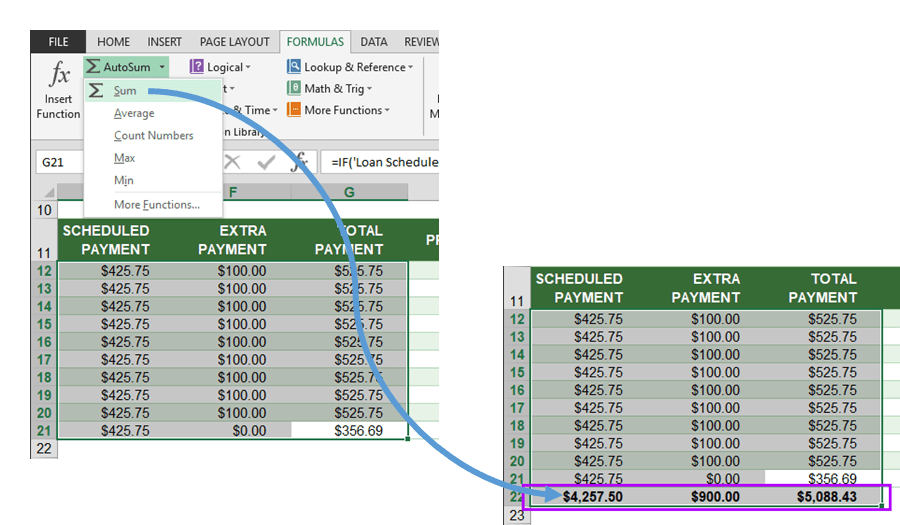Excel is more than a grid system labeled with coordinates. Sure, it can be used as a database or a simple calculator on your desktop, but its main advantage is the ability to create complex calculation models with little effort.

Formulas are the core component of Excel, with which logical statements can be written to manipulate data. By creating formulas, we can get the answers to anything from simple calculations to most complicated business problems. This doesn’t necessarily have to mean working only with numbers. Formulas can also manipulate text strings, copy a section to another, or extract certain portions for consumption in another section.

In this article, we’re going to be focusing on the use of the SUM formula. Let’s take a look at the structure of a formula, how it works, and how we can benefit from using it.

# Anatomy of a Formula

Formulas in Excel can contain any number of arithmetic operators, functions, references, and constants. Below is an example,Every formula starts with an equal sign. Next comes the function type. Functions are preset formulas that run calculations and return a value. In parentheses is a cell or range reference

return values of them as a single value or array of values. Also; Named Ranges can be used as references.

1. Operators: Symbols that contain characters for mathematical, logical, and set notation. Basically similar with mathematical operators.
2. Constants: Constant numerical or text values which can be entered directly. These values are static, so they won’t be affected by calculations.

# Creating a Formula

## 1. Manually Entering a Formula

Begin by selecting a cell by moving to it with your cursor or using the arrow keys on your keyboard, and type in an equal sign (“=” on the keyboard). Then, enter the function code and add a parenthesis. This is essentially the most common way to begin a formula.Keep in mind that you can use the Ctrl and Shift keys on your keyboard when selecting individual cells to define multiple target cells or ranges (i.e. A1, B3, HI589).

## 2. Using the Insert Function Feature

If you don’t know what function to enter, an alternative way to create a formula is to choose from Excel’s directory and add it to your model. This approach is especially useful if you’re not familiar with Excel’s function codes. To do this, click Insert Function button under the FORMULAS tab. You can also do this by pressing the fx button next to the formula bar.This will open the Insert Function window where you can see available functions with brief descriptions of what they do. You can also type in the functionality you’re looking for and press Go to look for a function that suits your need.Let’s take an example. The SUM function is used to add a set of cells together, essentially creating a ‘sum’ of those cells. To insert a SUM function using the Insert Function window follow these steps,

1. Select Math & Trig from the category drop-down.
2. Select SUM in function list.
3. Click OK to open Function Arguments4. Select cell/range arguments by clicking the input icon.
5. Click OK to apply function into the selected cell.## 3. Creating a Formula Directly From the Ribbon

Functions can be found in a categorized manner under the FORMULAS ribbon. Here, you can choose from a category, and then select the formula you want to use from the dropdown. This method essentially skips the Insert Function step, and jumps you right into the Formula Arguments window.For example, the SUM function can be found under Math & Trig category. Pressing this category, you will find the SUM function in the dropdown.

## 4. Using the AutoSum Feature to Sum Up Values

You can run some basic math operations without even entering a formula by using the AutoSum feature. The AutoSum menu can be found under the FORMULAS ribbon. For operations like taking a sum, average, count, maximum, or minimum of a set of values, this is one of the easiest methods.Selecting one will insert the corresponding function into the selected cell. AutoSum is a smart feature as it can predict the range you want to use in the function, and automatically places the function you choose. There are 2 ways to makes things easier for Excel to predict your selection,

Select data

Begin by selecting the range of cells you want to use for this operation, and click the function under AutoSum list. Make sure the cells below your selection are empty.Select cells below the data

You can achieve same result by only selecting cells just below your target data. Select the cells, and click the function under AutoSum list.Excel’s unprecedented flexibility offers users a sandbox tool that can capture, scrub, and process data. Formulas are the backbone of this software. Knowing how to use them effectively means that you’re going to be able to quickly analyze data and make sense of it. Using formulas in conjunction, it’s extremely easy to automate your calculations and data tasks.